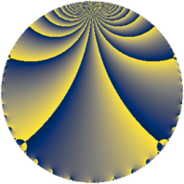# Properties

 Label 4864.2.a.bkLevel $4864$ Weight $2$ Character orbit 4864.a Self dual yes Analytic conductor $38.839$ Analytic rank $0$ Dimension $4$ CM no Inner twists $2$

# Learn more about

## Newspace parameters

 Level: $$N$$ $$=$$ $$4864 = 2^{8} \cdot 19$$ Weight: $$k$$ $$=$$ $$2$$ Character orbit: $$[\chi]$$ $$=$$ 4864.a (trivial)

## Newform invariants

 Self dual: yes Analytic conductor: $$38.8392355432$$ Analytic rank: $$0$$ Dimension: $$4$$ Coefficient field: $$\Q(\sqrt{3}, \sqrt{19})$$ Defining polynomial: $$x^{4} - 11 x^{2} + 16$$ Coefficient ring: $$\Z[a_1, \ldots, a_{7}]$$ Coefficient ring index: $$2$$ Twist minimal: no (minimal twist has level 1216) Fricke sign: $$-1$$ Sato-Tate group: $\mathrm{SU}(2)$

## $q$-expansion

Coefficients of the $$q$$-expansion are expressed in terms of a basis $$1,\beta_1,\beta_2,\beta_3$$ for the coefficient ring described below. We also show the integral $$q$$-expansion of the trace form.

 $$f(q)$$ $$=$$ $$q + \beta_{1} q^{5} + \beta_{2} q^{7} -3 q^{9} +O(q^{10})$$ $$q + \beta_{1} q^{5} + \beta_{2} q^{7} -3 q^{9} + ( 2 + \beta_{3} ) q^{11} + ( \beta_{1} - \beta_{2} ) q^{13} + \beta_{3} q^{17} - q^{19} + ( \beta_{1} + \beta_{2} ) q^{23} + ( 1 + \beta_{3} ) q^{25} + ( \beta_{1} - \beta_{2} ) q^{29} -2 \beta_{1} q^{31} + ( -2 + \beta_{3} ) q^{35} + ( 3 \beta_{1} + \beta_{2} ) q^{37} + ( 2 - 2 \beta_{3} ) q^{41} + ( 6 - \beta_{3} ) q^{43} -3 \beta_{1} q^{45} + \beta_{2} q^{47} + ( 3 - 3 \beta_{3} ) q^{49} + ( 3 \beta_{1} + 3 \beta_{2} ) q^{53} + ( 5 \beta_{1} + 2 \beta_{2} ) q^{55} -2 \beta_{3} q^{59} -\beta_{1} q^{61} -3 \beta_{2} q^{63} + 8 q^{65} + 2 \beta_{3} q^{67} + ( -4 \beta_{1} + 2 \beta_{2} ) q^{71} + \beta_{3} q^{73} + ( \beta_{1} - 2 \beta_{2} ) q^{77} + ( -4 \beta_{1} - 2 \beta_{2} ) q^{79} + 9 q^{81} + ( -4 - 4 \beta_{3} ) q^{83} + ( 3 \beta_{1} + 2 \beta_{2} ) q^{85} + 10 q^{89} + ( -12 + 4 \beta_{3} ) q^{91} -\beta_{1} q^{95} + ( 10 + 2 \beta_{3} ) q^{97} + ( -6 - 3 \beta_{3} ) q^{99} +O(q^{100})$$ $$\operatorname{Tr}(f)(q)$$ $$=$$ $$4q - 12q^{9} + O(q^{10})$$ $$4q - 12q^{9} + 6q^{11} - 2q^{17} - 4q^{19} + 2q^{25} - 10q^{35} + 12q^{41} + 26q^{43} + 18q^{49} + 4q^{59} + 32q^{65} - 4q^{67} - 2q^{73} + 36q^{81} - 8q^{83} + 40q^{89} - 56q^{91} + 36q^{97} - 18q^{99} + O(q^{100})$$

Basis of coefficient ring in terms of a root $$\nu$$ of $$x^{4} - 11 x^{2} + 16$$:

 $$\beta_{0}$$ $$=$$ $$1$$ $$\beta_{1}$$ $$=$$ $$\nu$$ $$\beta_{2}$$ $$=$$ $$($$$$\nu^{3} - 9 \nu$$$$)/2$$ $$\beta_{3}$$ $$=$$ $$\nu^{2} - 6$$
 $$1$$ $$=$$ $$\beta_0$$ $$\nu$$ $$=$$ $$\beta_{1}$$ $$\nu^{2}$$ $$=$$ $$\beta_{3} + 6$$ $$\nu^{3}$$ $$=$$ $$2 \beta_{2} + 9 \beta_{1}$$

## Embeddings

For each embedding $$\iota_m$$ of the coefficient field, the values $$\iota_m(a_n)$$ are shown below.

For more information on an embedded modular form you can click on its label.

Label $$\iota_m(\nu)$$ $$a_{2}$$ $$a_{3}$$ $$a_{4}$$ $$a_{5}$$ $$a_{6}$$ $$a_{7}$$ $$a_{8}$$ $$a_{9}$$ $$a_{10}$$
1.1
 −3.04547 −1.31342 1.31342 3.04547
0 0 0 −3.04547 0 −0.418627 0 −3.00000 0
1.2 0 0 0 −1.31342 0 4.77753 0 −3.00000 0
1.3 0 0 0 1.31342 0 −4.77753 0 −3.00000 0
1.4 0 0 0 3.04547 0 0.418627 0 −3.00000 0
 $$n$$: e.g. 2-40 or 990-1000 Significant digits: Format: Complex embeddings Normalized embeddings Satake parameters Satake angles

## Atkin-Lehner signs

$$p$$ Sign
$$2$$ $$-1$$
$$19$$ $$1$$

## Inner twists

Char Parity Ord Mult Type
1.a even 1 1 trivial
8.d odd 2 1 inner

## Twists

By twisting character orbit
Char Parity Ord Mult Type Twist Min Dim
1.a even 1 1 trivial 4864.2.a.bk 4
4.b odd 2 1 4864.2.a.bj 4
8.b even 2 1 4864.2.a.bj 4
8.d odd 2 1 inner 4864.2.a.bk 4
16.e even 4 2 1216.2.c.i 8
16.f odd 4 2 1216.2.c.i 8

By twisted newform orbit
Twist Min Dim Char Parity Ord Mult Type
1216.2.c.i 8 16.e even 4 2
1216.2.c.i 8 16.f odd 4 2
4864.2.a.bj 4 4.b odd 2 1
4864.2.a.bj 4 8.b even 2 1
4864.2.a.bk 4 1.a even 1 1 trivial
4864.2.a.bk 4 8.d odd 2 1 inner

## Hecke kernels

This newform subspace can be constructed as the intersection of the kernels of the following linear operators acting on $$S_{2}^{\mathrm{new}}(\Gamma_0(4864))$$:

 $$T_{3}$$ $$T_{5}^{4} - 11 T_{5}^{2} + 16$$ $$T_{7}^{4} - 23 T_{7}^{2} + 4$$ $$T_{11}^{2} - 3 T_{11} - 12$$

## Hecke characteristic polynomials

$p$ $F_p(T)$
$2$ $$T^{4}$$
$3$ $$T^{4}$$
$5$ $$16 - 11 T^{2} + T^{4}$$
$7$ $$4 - 23 T^{2} + T^{4}$$
$11$ $$( -12 - 3 T + T^{2} )^{2}$$
$13$ $$256 - 44 T^{2} + T^{4}$$
$17$ $$( -14 + T + T^{2} )^{2}$$
$19$ $$( 1 + T )^{4}$$
$23$ $$( -12 + T^{2} )^{2}$$
$29$ $$256 - 44 T^{2} + T^{4}$$
$31$ $$256 - 44 T^{2} + T^{4}$$
$37$ $$64 - 92 T^{2} + T^{4}$$
$41$ $$( -48 - 6 T + T^{2} )^{2}$$
$43$ $$( 28 - 13 T + T^{2} )^{2}$$
$47$ $$4 - 23 T^{2} + T^{4}$$
$53$ $$( -108 + T^{2} )^{2}$$
$59$ $$( -56 - 2 T + T^{2} )^{2}$$
$61$ $$16 - 11 T^{2} + T^{4}$$
$67$ $$( -56 + 2 T + T^{2} )^{2}$$
$71$ $$28224 - 348 T^{2} + T^{4}$$
$73$ $$( -14 + T + T^{2} )^{2}$$
$79$ $$3136 - 188 T^{2} + T^{4}$$
$83$ $$( -224 + 4 T + T^{2} )^{2}$$
$89$ $$( -10 + T )^{4}$$
$97$ $$( 24 - 18 T + T^{2} )^{2}$$
show more
show less